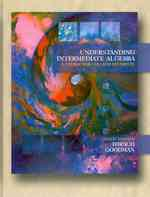### Understanding Intermediate Algebra : A Course for College Students; Annotated Instructor's Edition （6TH）

• ただいまウェブストアではご注文を受け付けておりません。 ⇒古書を探す
• 製本 Hardcover:ハードカバー版／ページ数 790 p.
• 言語 ENG,ENG
• 商品コード 9780495109020
• DDC分類 512

### Table of Contents

`    1 The Fundamental Concepts                     1  (64)      1.1 Basic Definitions: The Real Numbers      2  (12)      and the Real Number Line      1.2 Operations with Real Numbers             14 (15)      1.3 Algebraic Expressions                    29 (8)      1.4 Translating Phrases and Sentences        37 (10)      into Algebraic Form      1.5 First-Degree Equations and               47 (18)      Inequalities        Chapter 1 Summary                          57 (2)        Chapter 1 Review Exercises                 59 (3)        Chapter 1 Practice Test                    62 (3)    2 Equations and Inequalities                   65 (54)      2.1 Equations as Mathematical Models         66 (9)      2.2 First-Degree Equations and               75 (13)      Applications      2.3 First-Degree Inequalities and            88 (15)      Applications      2.4 Absolute-Value Equations and             103(16)      Ineqalities        Chapter 2 Summary                          112(3)        Chapter 2 Review Exercises                 115(3)        Chapter 2 Practice Test                    118(1)    3 Graphing Straight Lines and Functions        119(80)      3.1 The Rectangular Coordinate System and    120(12)      Graphing Straight Lines      3.2 Graphs and Equations                     132(12)      3.3 Relations and Functions: Basic           144(17)      Concepts      3.4 Function Notation                        161(8)      3.5 Interpreting Graphs                      169(30)        Chapter 3 Summary                          182(3)        Chapter 3 Review Exercises                 185(5)        Chapter 3 Practice Test                    190(2)        Chapter 1-3 Cumulative Review              192(4)        Chapter 1-3 Cumulative Practice Test       196(3)    4 Equations of a Line and Linear Systems in    199(64)    Two Variables      4.1 Straight Lines and Slope                 200(19)      4.2 Equations of a Line and Linear           219(14)      Functions as Mathematical Models      4.3 Linear Systems in Two Variables          233(16)      4.4 Graphing Linear Inequalities in Two      249(14)      Variables        Chapter 4 Summary                          255(3)        Chapter 4 Review Exercises                 258(3)        Chapter 4 Practice Test                    261(2)    5 Polynomial Expressions and Functions         263(56)      5.1 Polynomial Functions as Mathematical     264(7)      Models      5.2 Polynomial: Sums, Differences, and       271(6)      Products      5.3 General Forms and Special Products       277(9)      5.4 Factoring Out the Greatest Common        286(4)      Factor      5.5 Factoring Trinomials                     290(10)      5.6 Solving Polynomial Equations by          300(9)      Factoring      5.7 Polynomial Division                      309(10)        Chapter 5 Summary                          313(1)        Chapter 5 Review Exercises                 314(2)        Chapter 5 Practice Test                    316(3)    6 Rational Expressions and Functions           319(68)      6.1 Rational Functions                       320(5)      6.2 Equivalent Fractions                     325(5)      6.3 Multiplication and Division of           330(4)      Rational Expressions      6.4 Sums and Differences of Rational         334(8)      Expressions      6.5 Mixed Operations and Complex Fractions   342(6)      6.6 Fractional Equations and Inequalities    348(7)      6.7 Literal Equations                        355(4)      6.8 Applications: Rational Functions and     359(28)      Equations as Mathematical Models        Chapter 6 Summary                          374(2)        Chapter 6 Review Exercises                 376(4)        Chapter 6 Practice Test                    380(1)        Chapter 4-6 Cumulative Review              381(3)        Chapter 4-6 Cumulative Practice Test       384(3)    7 Exponents and Radicals                       387(68)      7.1 Natural Number and Integer Exponents     388(12)      7.2 Scientific Notation                      400(6)      7.3 Rational Exponents and Radical           406(11)      Notation      7.4 Simplifying Radical Expressions          417(8)      7.5 Adding and Subtracting Radical           425(3)      Expressions      7.6 Multiplying and Dividing Radical         428(5)      Expressions      7.7 Radical Functions and Equations          433(8)      7.8 Complex Numbers                          441(14)        Chapter 7 Summary                          448(3)        Chapter 7 Review Exercises                 451(3)        Chapter 7 Practice Test                    454(1)    Chapter 8 Quadratic Functions and Equations    455(94)      8.1 Quadratic Functions as Mathematical      456(7)      Models      8.2 Solving Quadratic Equations: The         463(13)      Factoring and Square Root Methods      8.3 Solving Quadratic Equations:             476(5)      Completing the Square      8.4 Solving Quadratic Equations: The         481(7)      Quadratic Formula      8.5 Equations Reducible to Quadratic Form    488(10)      (and More Radical Equations)      8.6 Graphing Quadratic Functions             498(19)      8.7 Quadratic and Rational Inequalities      517(10)      8.8 The Distance Formula: Circles            527(22)        Chapter 8 Summary                          538(5)        Chapter 8 Review Exercises                 543(5)        Chapter 8 Practice Test                    548(1)    9 More on Functions                            549(50)      9.1 More on Function Notation: Split         550(7)      Functions      9.2 Composition and the Algebra of           557(6)      Functions      9.3 Types of Functions                       563(7)      9.4 Inverse Functions                        570(8)      9.5 Variation                                578(21)        Chapter 9 Summary                          586(4)        Chapter 9 Review Exercises                 590(2)        Chapter 9 Practice Test                    592(2)        Chapter 7-9 Cumulative Review              594(3)        Chapter 7-9 Cumulative Practice Test       597(2)    10 Exponential and Logarithmic Functions       599(50)      10.1 Exponential Functions                   600(10)      10.2 Logarithms and Logarithmic Functions    610(7)      10.3 Properties of Logarithms                617(5)      10.4 Common Logarithms, Natural              622(5)      Logarithms, and Change of Base      10.5 Exponential and Logarithmic Equations   627(7)      10.6 Applications: Exponential and           634(15)      Logarithmic Functions as Mathematical      Models        Chapter 10 Summary                         643(2)        Chapter 10 Review Exercises                645(2)        Chapter 10 Practice Test                   647(2)    11 More Systems of Equations and Systems of    649(72)    Inequalities      11.1 3x3 Linear Systems                      650(8)      11.2 Solving Linear Systems Using            658(10)      Augmented Matrices      11.3 The Algebra of Matrices                 668(9)      11.4 Solving Linear Systems Using Matrix     677(9)      Inverses      11.5 Determinants and Cramer's Rule          686(8)      11.6 Systems of Linear Inequalities          694(7)      11.7 Nonlinear Systems of Equations          701(20)        Chapter 11 Summary                         708(5)        Chapter 11 Review Exercises                713(2)        Chapter 11 Practice Test                   715(2)        Chapter 10-11 Cumulative Review            717(2)        Chapter 10-11 Cumulative Practice Test     719(2)  Appendixes      A Sets                                       721(6)      B Conic Sections                             727(20)      C Sequences and Series: The Binomial         747      Theorem        C.1 Sequences                              747(6)        C.2 Series and Sigma Notation              753(4)        C.3 Arithmetic Sequences and Series        757(9)        C.4 Geometric Sequences and Series         766(11)        C.5 The Binomial Theorem                   777(8)        Appendix C Summary                         785(2)        Appendix C Review Exercises                787(2)        Appendix C Practice Test                   789Answers to Selected Exercises and Chapter Tests    1  (1)Index                                              1`